# Speed of sound

The average speed of sound is 330 meters per second. Estimate how long it will take to hear the church bell 1 km away. Calculate the distance from what would hear sound after 10 seconds.

t =  3.0303 s
x =  3.3 km

### Step-by-step explanation: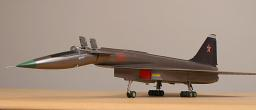Did you find an error or inaccuracy? Feel free to write us. Thank you!Tips to related online calculators
Do you want to convert length units?
Do you want to convert velocity (speed) units?
Do you want to convert time units like minutes to seconds?

## Related math problems and questions:

• Thunder and lightning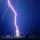There was a glimpse of the sky, and we hear thunder in 4.5 seconds. The light spreads at 300,000 kilometers per second so that we can assume the flash instantly without delay. However, the sound's speed is much lower in the air, one-third of a kilometer p
• Speeds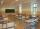George pass on the way to school distance 200 meters in 165 seconds. What is the average walking speed in m/s and km/h?
• Two trains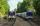Two trains go at each other. First at speed 40 km/h and the second at speed 60 km/h. At what distance will be 30 minutes before they will meet?
• Two citiesThe distance between cities A and B is 132 km. At 9.00 am, the cyclist started the bike at an average speed of 24 km/h, and at 10.00 am started from the B cyclist at an average speed of 30 km/h. How long and far from A will they both meet?The railroad runs parallel to the railway. The train travels at 36 km/h on track. In the opposite direction, the motorcycle rides at 90 km/h. The train passes in 3 seconds. How long is a train?
• Fog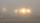The car started in fog at a speed of 30 km/h. After a 12-minute drive, the fog dissipated, and the driver drove the next 12 minutes distance, 17 km. On the last 17 km long again, the driving conditions deteriorated, and the driver drove at a speed of 51 k
• Reconstruction of the corridorCalculate how many minutes will be reduced to travel 187 km long railway corridor, where the maximum speed increases from 120 km/h to 160 km/h. Calculate how many minutes will shorten travel time, if we consider that the train must stop at 6 stations, eac
• Athletic competitionIn a 400 meter athletic competition, a participant covers the distance as given below. find the average speed? first 80 meters 10 m/s next 240 meters 7.5 m/s last 80 meters 10 m/s
• Train speedTwo guns were fired from the same place at an interval of 10 minutes and 30 seconds, but a person in a train approaching the place hears second shot 10 minutes after the first. The speed of the train (in km/hr), supposing that sound travels at 340 m/s is: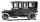Car started at 11:40 at speed 54 km/h from A and to B arrived at 12:20. Determine the distance A and B.
• Walking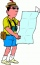The boy walked about 8.5 km in an hour. How long will it take to walk a distance of 32 km if he takes two breaks of 30 minutes during the route?
• Cheetah vs antelope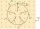When the cheetah began chasing the antelope, the distance between them was 120 meters. Although the antelope was running at 72km/h, the cheetah caught up with it in 12 seconds. What speed was the cheetah running?
• Sound speed in Earth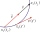Through the surface layers of the Earth, sound propagates 13 times faster than through air. During the geological survey, the charge exploded 1.7 km from the measuring station. How long does it take between the vibration of the soil at the measuring point
• I drive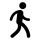I drive 9 km in 30 minutes, what is the average speed of the motion?
• Constant motionThe car has undergone constant motion 120 km in 1 hour 30 minutes. Determine its average speed. Determine how long the car drove in the first 20 minutes and 50 minutes from the start of the move.
• Two shipsThe distance from A to B is 300km. At 7 am started from A to B a ferry with speed higher by 20 km/h than a ship that leaves at 8 o'clock from B to A. Both met at 10h 24min. Determine how far they will meet from A and when they reach the destination.
• Motion against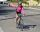From two places 36 km apart, a pedestrian and a cyclist will go straight opposite each other. How long will they meet if the speed of the pedestrian is 4 km/h and the cyclist 12 km/h?